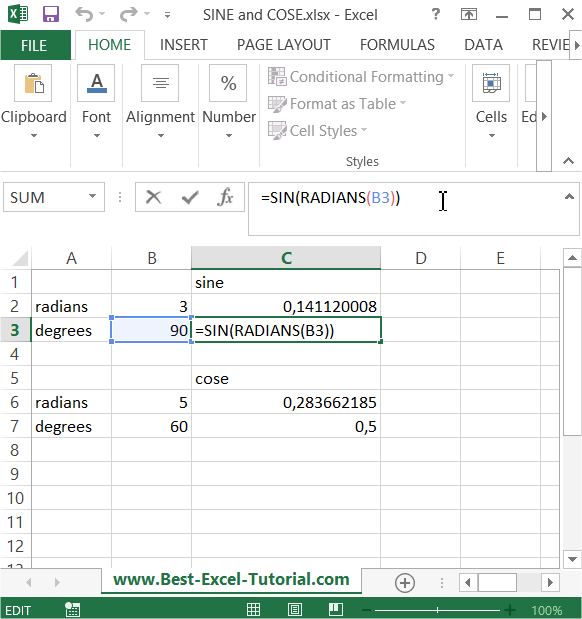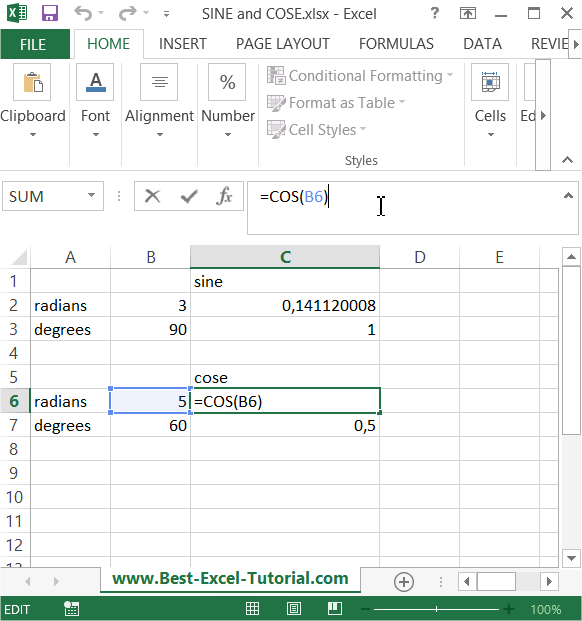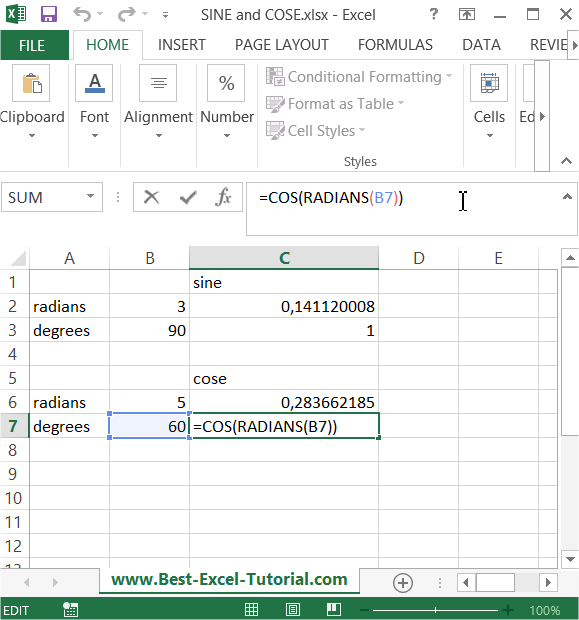#### How to Use Trig Functions in Excel?

Trigonometry is a branch of mathematics that studies the relationships between the elements (sides and angles) of a triangle.

You may recall many trigonometric formulas and equations from your school or college days. Some of them are cot x = 1/tanx , six x/cos x = tan x, sin(900-x) – cos x and so on.

Excel offers a number of built-in functions that deal with trigonometry. You can use these trig functions to solve complex trigonometric expressions.

The main thing you need to consider while solving trigonometric expressions is that Excel performs the calculations considering angle values in radians and not in degrees. You might know that sin 900 = 1. So, if you enter the formula SIN (90) in Excel, the result will be .893997 and not 1, because Excel considers 90 as 90 radians and not 90 degrees.

If you want to find the sine of 90 degrees, then you should first convert degrees into radians and then use the SIN formula available in Excel. Don’t worry, we are going to look at how to use trigonometric functions in Excel in a few minutes.

Excel provides functions for sine (sin), cosine (cos), tangent (tan), hyperbolic sine (sinh), hyperbolic cosine (cosh), and hyperbolic tangent (tanh).

Excel does not provide functions for secant (sec), cosecant (cosec), cotangent (cot) and their hyperbolic counterparts. However, you can calculate these functions using the core functions (sine and cosine). Excel also offers functions to convert angles from radians to degrees and vice versa.

## Using Trigonometric Functions in Excel

Open Excel and save your file as trig-functions.xlsx. Type “Angle (Degrees)” in A1, “Angle (Radians)” in B1, “SIN” in C1, “COS” in D1, “TAN” in E1, “COSEC” in F1, “SEC” in G1 and “COT” in H1. Also type “0” in A2, “30” in A3, “45” in A4, “60” in A5, “90” in A6, “180” in A7, “270” in A8 and “360” in A9. You should not enter the double quotes when you type in the data. You can format this text and make it bold.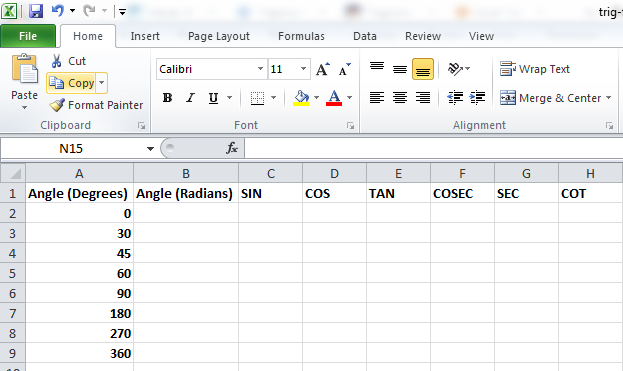Click on cell B2 and go to Formulas (main menu) –> Math & Trig (in the Function Library group).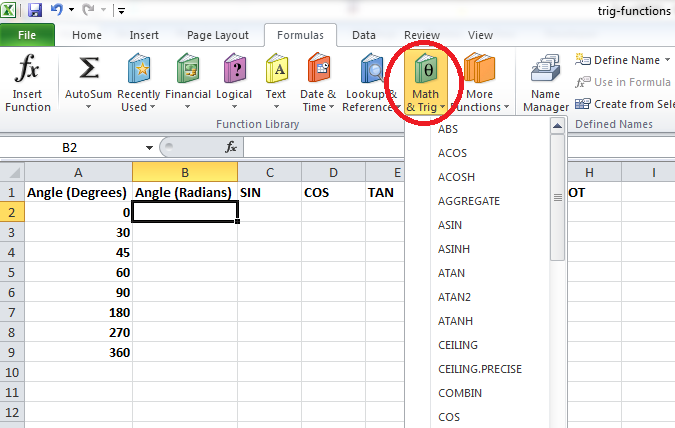Scroll down and select the RADIANS function to get a screen like this: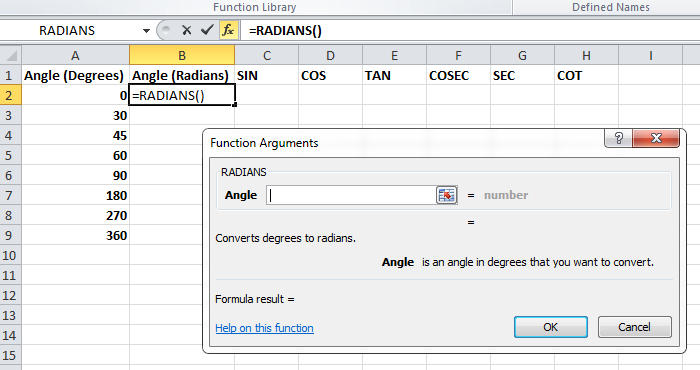After clicking inside the space to enter the value (circled in red), click the cell A2.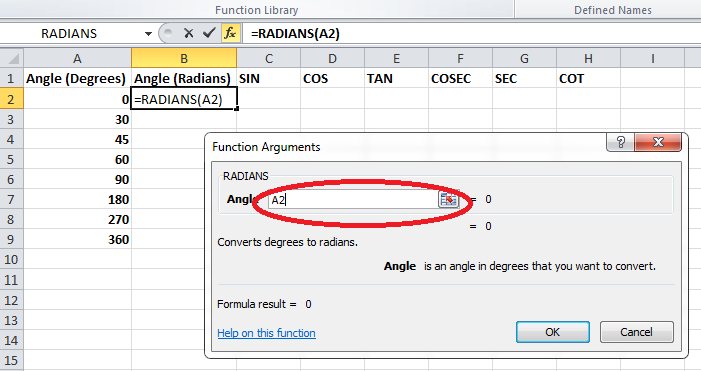Click OK and the cell B2 will have a value of 0.

Click on cell B2 and copy the formula (CTRL + C) and paste it (CTRL + V) in the cells B3, B4, B5, B6, B7, B8 and B9. If you are an experienced Excel user, you can just drag the formula into the cells instead of copying and pasting.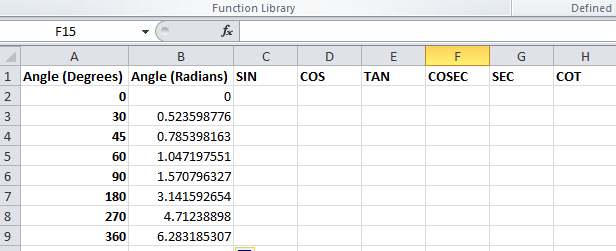Click on cell C2 and go to Formulas –> Math & Trig (in the Function Library group). Select the SIN function and after clicking inside the space to enter the value, click on cell B2. Click OK. Copy the formula from cell C2 and paste it into the cells C3 to C9.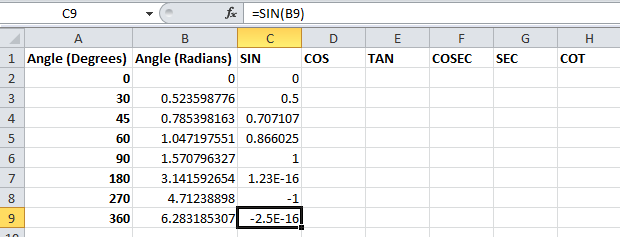Click on cell D2 and go to Formulas –> Math & Trig (in the Function Library group). Select the COS function and after clicking inside the space to enter the value, click on cell B2. Click OK. Copy the formula from cell D2 and paste it into the cells D3 to D9.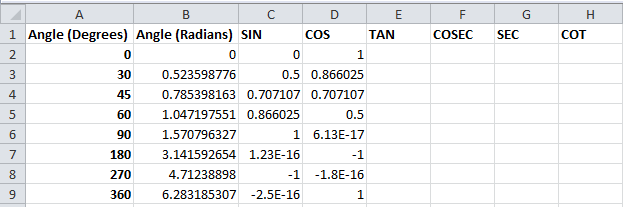Click on cell E2 and go to Formulas –> Math & Trig (in the Function Library group). Select the TAN function and after clicking inside the space to enter the value, click on cell B2. Click OK. Copy the formula from cell E2 and paste it into the cells E3 to E9.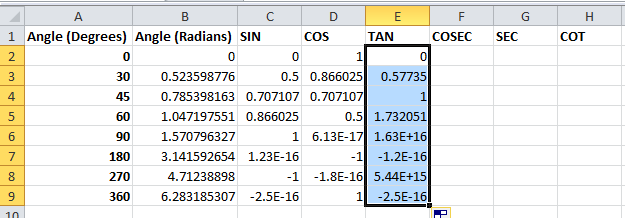As already mentioned, there are no built-in functions to calculate COSEC, SEC and COT values. You need to calculate them using the core functions like this:

cosec x = 1/sin x

sec x = 1/cos x

cot x = 1/tan x

Click on cell F2 and click inside the Formula Bar (circled in red) and enter the formula “=1/C2” (without double quotes). Copy the formula in cell F2 and paste it into the cells F3 to F9.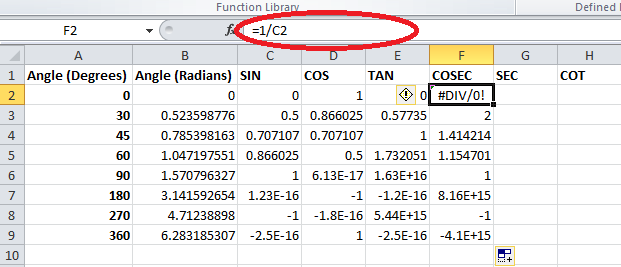Click on cell G2, click inside the Formula Bar and enter the formula “=1/D2” (without double quotes). Copy the formula in cell G2 and paste it into the cells G3 to G9.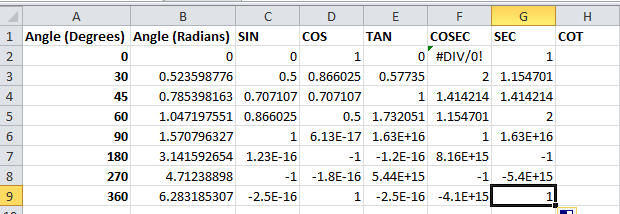Click on cell H2, click inside the Formula Bar, and enter the formula “=1/E2” (without double quotes). Copy the formula in cell H2 and paste it into the cells H3 to H9.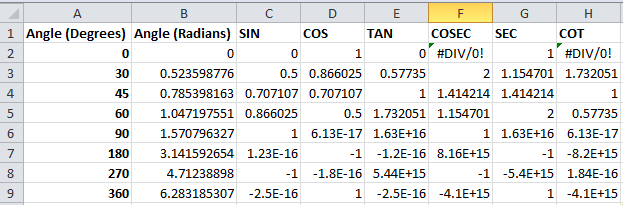You can round these resulting values to two or three decimal points to get more feasible results. Change all the formulas in cells C, D, E, F, G and H in such a way that the new formula becomes =ROUND(existing formula, 3). For example, the formula in cell C4 becomes =ROUND(SIN(B4),3) where the existing formula was =SIN(B4).

You can also replace all the errors (#DIV/0!) with * and just provide a description somewhere in the same worksheet specifying that * means undefined.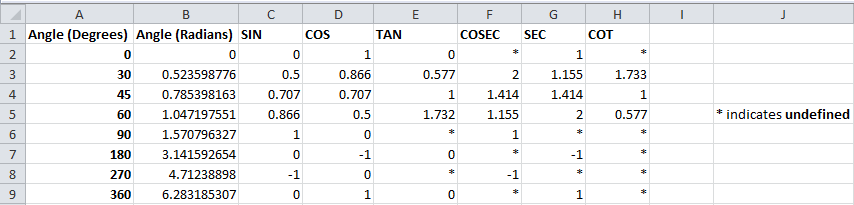Likewise, you can find the value of sinh, cosh and tanh using the formulas SINH, COSH and TANH and calculate cosech, sech and coth from sinh, cosh and tanh.

## Calculating sine and cosine of an angle

Here you will see how to calculate sine and cosine when you have radians or degrees. To calculate sine of an angle you can just use Excel SIN function. Formula is =SIN(radians)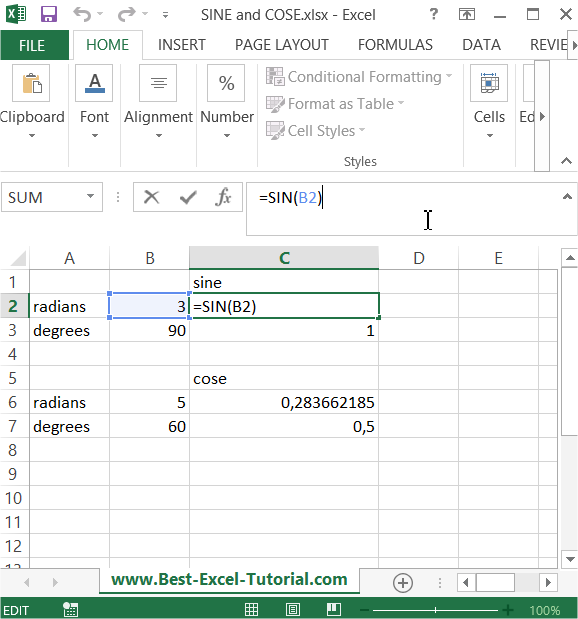But what to do when you know only degrees? There is also Excel RADIANS function built-in =RADIANS(degrees) which convert degrees to radians. Just use it. Formula for sine with degrees is =SIN(RADIANS(degrees))